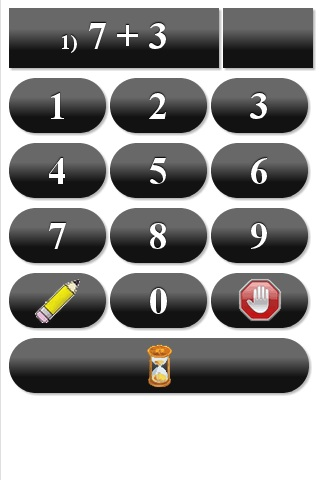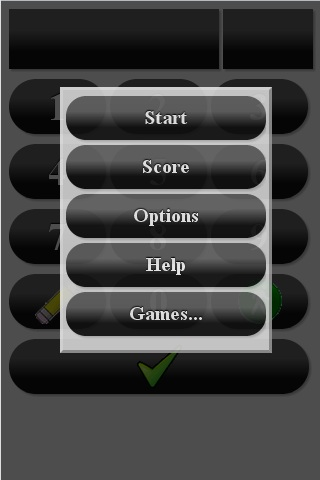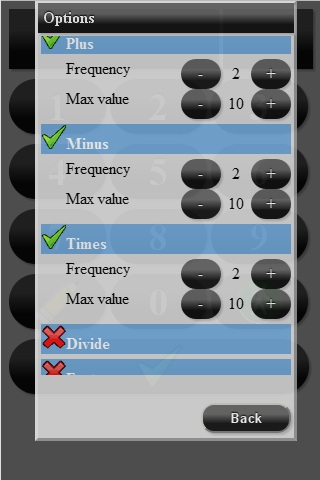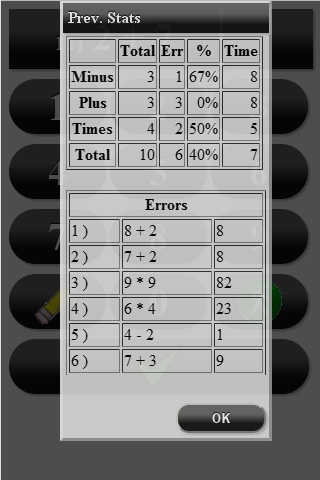﻿ Math Tutor | GASP Mobile Games

# Math Tutor HomeTry Online
Tired of math tutoring that are more of a game than tutoring? You are at the right spot. We don't have cute Farmville pets or angry birds. Instead we are striving to build your 5-8 year old child elementary school level arithmetic fluency skills at the least time possible.
The parent can configure what type of math skills and at what level to exercise. Parent should instruct child how many problems to solve. Last test results (including total number, number of errors, percent correct and average time for each type of math operation) as well as incorrect answers are saved. The report is available and can be used by the parent to monitor child success. If number of errors or average time is unsatisfactory, parent can optionally instruct the child to take the test again.
Each mathematical operation Plus (addition), Minus (subtraction), Times (multiplication), Divide (division) and Factors (especially useful to prepare for notoriously difficult 60-second sweep) can be either opted out, or once selected can be configured for frequency. Plus, Minus and Times also can be configured for the Max-Value – maximum result for addition, or max first operand for subtraction and multiplication. This way, for example, the parent can configure the test for infrequent subtraction from numbers as large as 30 and more frequent multiplication by 2, 3 and 4, so the child still practices subtractions while starting to study multiplication table.
The Factors operation was specifically introduced to help children to master 60-seconds sweep where they need to know all the factors of the simple numbers – e.g. Factors of 8 is 2 and 4. When testing factors please instruct your child to enter all the factors of the number – Factors of 8=24, Factors of 12=2634 (if more than one pair the order doesn’t matter)..